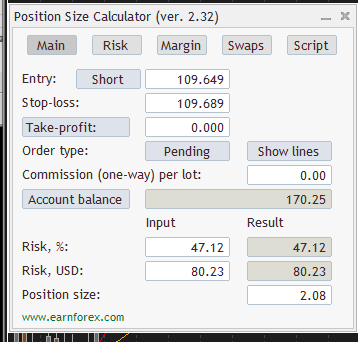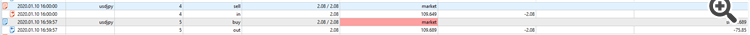# MQL5 function which calculates position size by risk and SL

Have tried searching and implementing but cannot find correct example. Its weird because it should be needed for lot of people and should exist somewhere.

Can you give a link or code for that?

So far I have done by examples this

``` double calculateLotSizeForPips(double slPips, double maxRiskPerTradePercent){          //Calculate the size of the position size
double LotSize=0;
//We get the value of a tick
//double nTickValue=MarketInfo(Symbol(),MODE_TICKVALUE); // not used

//If the digits are 3 or 5 we normalize multiplying by 10
/*if(_Digits==3 || _Digits==5){
nTickValue=nTickValue*10;
}*/

float balance = AccountInfoDouble(ACCOUNT_BALANCE);

//We apply the formula to calculate the position size and assign the value to the variable
//LotSize=MathRound(LotSize/MarketInfo(Symbol(),MODE_LOTSTEP))*MarketInfo(Symbol(),MODE_LOTSTEP); // not used

double volumeStep = SymbolInfoDouble(Symbol(),SYMBOL_VOLUME_STEP);
LotSize=MathRound(LotSize/volumeStep)*volumeStep;
return LotSize;
}```

But it works bad. Testing on USDJPY pair, usd account.

I do not know why in the example was when digits are 3 or 5 - multiply by ten , but it make my position 10 times smaller.

So for 10k account, when I put to formula it is:

Risk 2 percent, pips 4, tick value 26

10000*0.02/(4*26) = 1.92

Code with volume step is not changing the result.

But it is wrong.It loses about 80 usd. 2 % from 10k is 200.

Backtest shows it has lost about 75its more or less what the function calculated. But it is not 200.

Reason: